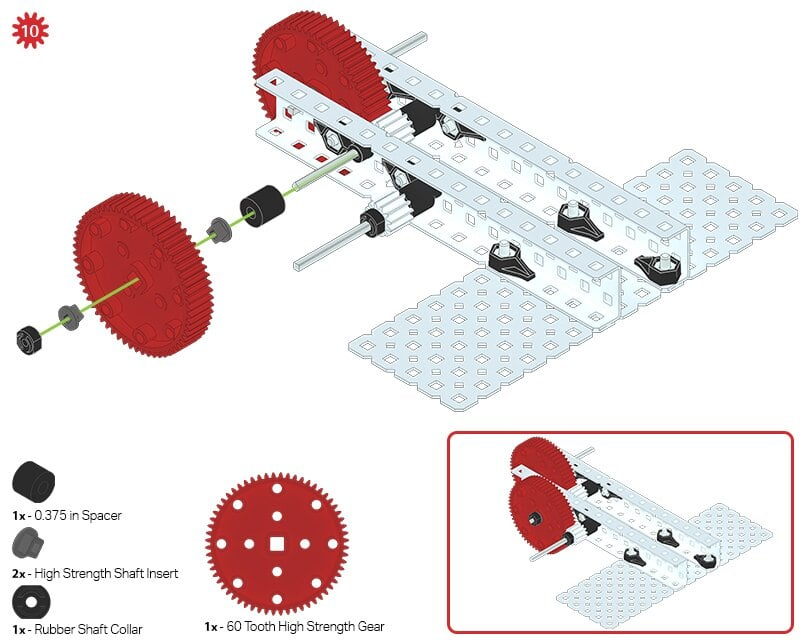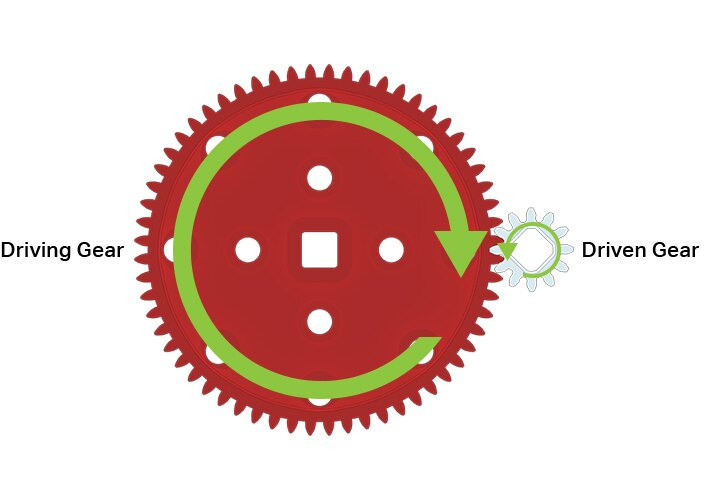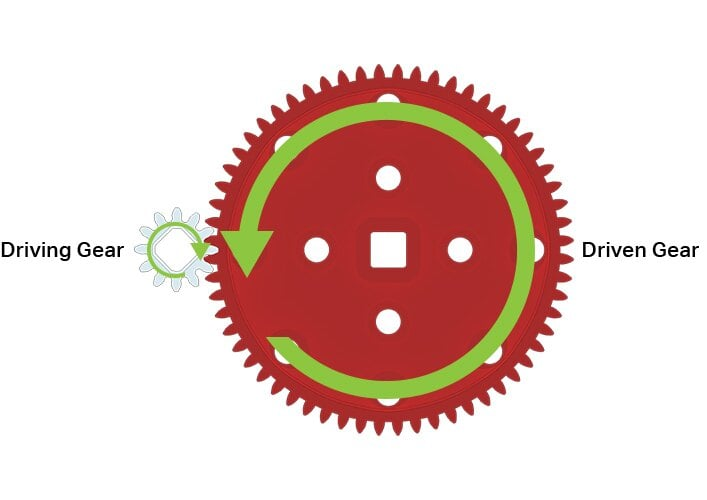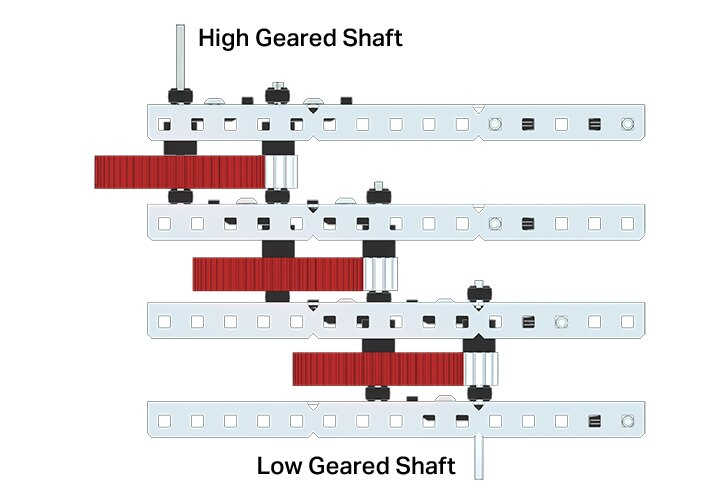STEMLabs V5

Teacher Portal

# Applying Gears to this Build

### Step 1: The Gears in a Single Layer of the V5 Gear Box BuildStep  10 of the V5 Gear Box build

In every layer of the gearbox, you meshed a 12-tooth gear with a 60-tooth gear. Notice that the 60-tooth gear is on the shaft that turns when you turn the shaft lock plate. When the 60-tooth gear turns, it turns the 12-tooth gear. That means that on this side of the gearbox, the 60-tooth gear is the driving gear and the 12-tooth gear is the driven gear. Locate the three locations in the build where a 60-tooth gear drives a 12-tooth gear. Hint: It matters which shaft you're turning. You can use a Shaft Collar extended to a wheel or another shaft-locked part to make it easier.

Since there are 5 times as many teeth on the larger gear, our gear ratio is 5:1, meaning every 5 turns of the smaller gear, the larger gear will turn 1 time.Teacher Toolbox

Students are asked to locate the three locations where the 60-tooth gear drives a 12-tooth gear. The 60-tooth gears are easy to recognize in the build. Every 60-tooth gear in the build drives a 12-tooth gear, but only when you are turning the shaft that turns the 60-tooth gear. The other shaft turns the 12-tooth gear which then drives the 60-tooth gear.

### Step 2: Using a Larger Gear as an Input for Increased SpeedUsing a 60-tooth gear to drive a 12-tooth gear

Using a 60-tooth gear to drive a 12-tooth gearational speed around an axle. In the example above, we are turning or driving the large gear. For each 1 turn around for the larger, driving gear, the smaller gear has to turn 5 whole times around. This means that the smaller gear will spin 5 times as fast as the larger gear, due to the 5:1 gear ratio. This is known as high gear ratio; the ratio is higher than 1:1.

### Step 3: Using a Smaller Gear as an Input for Increased TorqueUsing a 12-tooth gear to drive a 60-tooth gear

Torque is a measure of how hard you turn something about a center point. You can calculate it by multiplying the force of a push by how far away the push is from the center point. Since the teeth of the smaller, driving gear are close to the center, they push with more force for the same amount of torque. At the same time, the teeth of the driven gear are far away from the center point, so the same amount of force creates more torque.

If you have a larger driving gear, the torque you need to apply to the shaft is much higher than if you were driving a smaller gear. Since we can attach multiple gears to the same shaft, we can adjust the amount of force from the same torque, resulting higher torque with each link. The resultant gear ratio from each stage of our V5 Gear Box is 1:5, a low gear ratio. The driving gear will have to turn further to move the driven gear, but it will make the driven gear push harder.Motivate Discussion

The previous two steps provided important information. This discussion should serve as a simplified recap of that information.

Q: When the driving gear is larger than the driven gear, does the driven gear have more speed or more torque? Why?
A: The driven gear has more speed because it spins multiple times during a single rotation of the larger driving gear.

Q: When the driving gear is smaller than the driven gear, does the driven gear have more speed or more torque? Why?
A: The driven gear has more torque because the driven gear's teeth are farther away from its center point than the driving gear's teeth are from its center point.

### Step 4: Multiplying Mechanical AdvantageThe gearbox, showing high and low geared shafts.

Each stage has a 5:1 gear ratio, so we can multiply them together to make 5x5x5:1x1x1, or 125:1 ratio between input and output shafts. That means that if you turn the high-geared shaft 1 time all the way around, the output shaft will turn 125 times! On the other hand, if you apply some torque to the low-geared shaft, the output shaft will output with a torque 125 times as high, but it will do it very slowly.

Try turning the high geared shaft with no resistance. How quickly does the output shaft turn?

Try turning the low geared shaft while holding the output shaft. Can you stop the output shaft from turning?Teacher Tips

• Instruct students to turn the low gear shaft. Some students may have trouble turning it because of the extreme amount of torque created by the compound gear ratio.

• ﻿Ask students to turn the high gear shaft. The result will be the low geared shaft spinning at a noticeably faster pace.

• Explain that this machine's behavior is due to the extreme compound gear ratio which is 125:1.

• Emphasize that compound gear ratios are commonly used when the required mechanical advantage is more than a single gear ratio can achieve.Extend Your Learning

To expand﻿ this activity, use the information within this lab and the formulas below to calculate output speed and output torque of a given gear ratio.

Formulas:
Gear Reduction = Driven Gear Teeth / Driving Gear Teeth
Output Torque = Input Torque x Gear Reduction
Output Speed = Input Speed / Gear Reduction

Use ratios that can be created within the VEX V5 Classroom Super Kit.

Example:
Gear Ratio = 60:12 or 5:1
Gear Reduction = Driven Gear Teeth / Driving Gear Teeth = 12 / 60 = 0.2
Output Torque = Input Torque x Gear Reduction = 1.5 N-m x 0.2 = 0.3 N-m
Output Speed = Input Speed / Gear Reduction = 100 RPM / 0.2 = 500 RPM